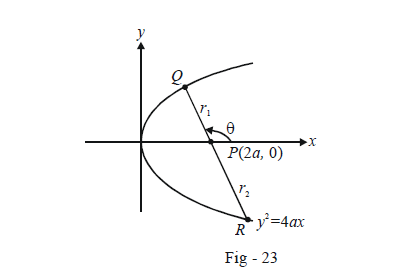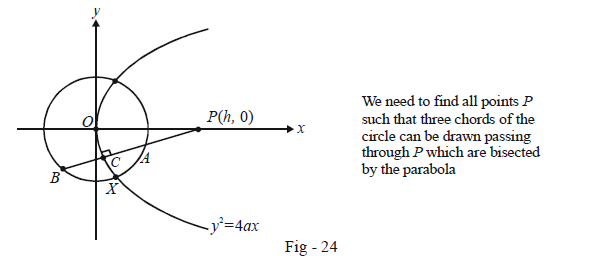# Basic Examples on Parabolas Set 4

Go back to  'Parabola'

Example - 14

Let P be the point (2a, 0) and QR be a variable chord of the parabola $${y^2} = 4ax$$ passing through P. Prove that

$\frac{1}{{P{Q^2}}} + \frac{1}{{P{R^2}}} = {\rm{constant}}$

Solution: Since distances are involved, we can use the polar form for a straight line to write the equation of QR. Let $$PQ{\text{ }} = {\text{ }}{r_1}\;and\;{\text{ }}PR{\text{ }} = {\text{ }}{r_2}$$.Also, let QR be at an inclination $$\theta$$The co-ordinates of any point on QR can be written as (in terms of its distance r from P) $$(2a + r\cos \theta ,\,\,r\sin \theta ).$$ If this lies on the parabola, we have

\begin{align}&\qquad{r^2}{\sin ^2}\theta = 4a(2a + r\cos \theta )\\&\!\!\! \Rightarrow \quad {r^2}{\sin ^2}\theta - 4ar\cos \theta - 8{a^2} = 0 \qquad \qquad\qquad \dots\left( 1 \right)\end{align}

Since Q and R lie on the parabola,$${r_1}\;and\;{r_2}$$ must satisfy (1).

Thus,

${r_1} + {r_2} = \frac{{4a\cos \theta }}{{{{\sin }^2}\theta }},\,\,\,{r_1}{r_2} = \frac{{ - 8{a^2}}}{{{{\sin }^2}\theta }}\qquad \qquad\qquad\dots \left( 2 \right)$

Finally,

\begin{align}&\frac{1}{{P{Q^2}}} + \frac{1}{{P{R^2}}} = \frac{1}{{r_1^2}} + \frac{1}{{r_2^2}}\\\\& \qquad \qquad\qquad= \frac{{r_1^2 + r_2^2}}{{{{({r_1}{r_2})}^2}}}\\\\&\qquad \qquad\qquad = \frac{{{{({r_1} + {r_2})}^2} - 2{r_1}{r_2}}}{{{{({r_1}{r_2})}^2}}}\\\\&\qquad \qquad\qquad=\frac{\begin{align}{\frac{16{{a}^{2}}{{\cos }^{2}}\theta }{{{\sin }^{4}}\theta }+\frac{16{{a}^{2}}}{{{\sin }^{2}}\theta }}\end{align}}{\begin{align}{\frac{64{{a}^{4}}}{{{\sin }^{4}}\theta }}\end{align}}\qquad (\text{using}\;(\text{2}))\\\\&\qquad \qquad\qquad= \frac{1}{{4{a^2}}} \end{align}

which is a constant.

Example – 15

Find all the points on the x-axis from which exactly three distinct chords of the circle $${x^2} + {y^2} = {a^2}$$ can be drawn which are bisected by the parabola $${y^2} = 4ax\,\,(a > 0).$$

Solution: Let such a point be P(h, 0). We need to find the possible values that h can take.Note that one such chord will always be simply along the x-axis because it is bisected by the parabola at the origin.

Referring to Fig - 24, let C be the point t so that its co-ordinates are $$(a{t^2},\,\,2at).$$ We can write the equation of the chord of the circle $${x^2} + {y^2} = {a^2}$$ bisected at C as

\begin{align}& \qquad \quad \,\,\, T(a{t^2},\,\,2at) = S(a{t^2},\,\,2at)\\&\Rightarrow \qquad tx + 2y = a{t^3} + 4at\end{align}

Since this passes through P(h, 0), we have

\begin{align}& \qquad \;\; th = a{t^3} + 4at\\& \Rightarrow \quad t(a{t^2} + (4a - h)) = 0 \qquad \dots \left( 1 \right)\end{align}

One of the roots of (1) is t = 0 which corresponds to the case already mentioned, the diameter along the x-axis.

The other two roots are real and distinct from zero if

\begin{align}& \qquad \;\frac{h}{a} - 4 > 0\\& \Rightarrow \quad h > 4a \qquad \qquad \dots \left( 2 \right)\end{align}

If you think carefully, you will realise that an additional constraint has to be imposed, namely, a limit on the value of t so that $$(a{t^2},2at)$$ lies inside the circle, since only then will a chord be formed. Thus,

\begin{align}& \qquad \quad \;\; {(a{t^2})^2} + {(2at)^2} < {a^2}\\& \Rightarrow \qquad {t^2} < \sqrt 5 - 2 \qquad \qquad\qquad\qquad \dots\left( 3 \right)\end{align}

From (1), we can see that (3) can equivalently be written as

\begin{align}& \qquad \;\frac{h}{a} - 4 < \sqrt 5 - 2\\&\Rightarrow \quad h < (\sqrt 5 + 2)\,a\end{align}

From (2) and (3), we see that the possible range of h is

$h \in \left( {4a,\left( {\sqrt 5 + 2} \right)a} \right)$

## TRY YOURSELF - I

Q. 1     Find the equation of the parabola with vertex (2, –3) and focus (0, 5).

Q. 2     Find the equation of the parabola with vertex at (2,1) and directrix $$x = y\; –1.$$

Q. 3     Find the locus of the middle points of all chords of the parabola $${y^2} = 4ax$$ which are drawn through the vertex.

Q. 4     Find the length of the side of the equilateral triangle inscribed in the parabola $${y^2} = 4ax$$ , with one vertex at the origin.

Q. 5     Find the locus of a point which divides a chord of slope 2 of the parabola $${y^2} = 4x$$ internally in the ratio 1 : 2.

Q. 6     Find the locus of the centre of the circle described on any focal chord of the parabola $${y^2} = 4ax$$ as diameter.

Q. 7     Find the locus of the mid-points of all chords of length C of the parabola $${y^2} = 4ax$$ .

Q. 8     Two chords of the parabola $${y^2} = 4ax$$  passing through its vertex are perpendicular to each other. If $${{p}_{1}}\,and\,{{p}_{2}}$$ be the lengths of these chords, prove that

${({p_1}{p_2})^4} = {(16{a^2}(p_1^{2/3} + p_2^{2/3}))^3}$

Q. 9      Consider a variable chord PQ through the focus of $${y^2} = 4ax$$ . The straight line joining P to the vertex cuts the line joining Q to the point (–a, 0) at R.
Find the locus of R.

Q. 10     From the vertex of the parabola $${y^2} = 4ax$$ , a pair of chords is drawn, perpendicular to each other. With those chords as adjacent sides a rectangle is completed.
Find the locus of the vertex of the rectangle opposite to the vertex of the parabola.